##### Actions

(diff) ← Older revision | Latest revision (diff) | Newer revision → (diff)

A notion in intuitionistic mathematics (see Intuitionism). It is a population, a species, consisting of finite sequences (cf. Tuple) of positive integers, called the nodes of the spread (or the admissible sequences of the spread). More precisely, a species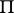of sequences of natural numbers is called a spread if the following conditions are satisfied: 1) there exists an effective rule(a so-called spread law) by which for any sequences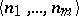one can determine whether or not it is a node of; 2) the empty sequence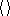is a node of any spread; 3) if the sequenceis a node of the spread, then any initial sequence of it, of the form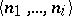for, is also a node of; and 4) if the sequenceis a node of, then there exists a positive integersuch that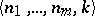is a node of.

If one orders the sequences of positive integers by considering thatif and only ifis a proper initial part of, then from the point of view of this order the spreadis an infinite tree with origin, given effectively (given by a law). A choice sequence(or, more generally, an arbitrary effective function converting natural numbers into natural numbers) is called an element of a spread, symbolically, if for anythe sequence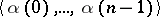is a node of. In applications one often has to deal with the notion of a labelled spread. A labelled spreadconsists of a spreadand an effective rule(the so-called complementary spread law) attributing to each nodeofsome object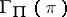. Thus there is a natural correspondence between each element of the spreadand a sequence of objects given by the law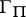.

In the language of formal intuitionistic mathematical analysis a spread is given by a function — its spread law. With that end in view one considers the standard primitive recursive one-to-one correspondence between sequences of natural numbers and natural numbers. Let the tuplecorrespond to, let the operation of concatenating two sequences be coded by a primitive recursive function, and let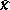denote the number of the sequence with unique element. The statement that the tuple with numberis a node of the spread given byis written as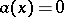. Then the formuladescribing the notion "the function a defines a spread" is written in the form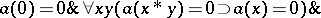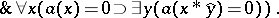Finally, if by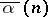one denotes the number of the sequence, whereis the length of the sequence, then the formula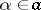( "a is an element of the spread given by a" ) is written as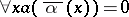.

In the foundations of mathematics, generalizations of the concept of a spread, in which sequences of natural numbers are replaced by sequences of more complicated objects (for example, by sequences consisting of choice sequences) are also considered.

How to Cite This Entry: